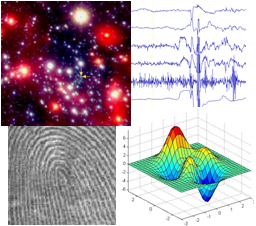CS 112 Assignment 1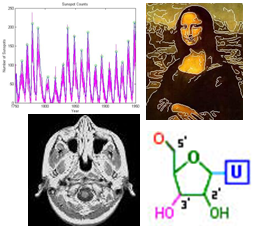## Due: Friday, February 13

You can turn in your assignment up until 5:00pm on 2/13/14. You should hand in both a hardcopy and electronic copy of your solutions. Your hardcopy submission should include printouts of four code files: ```golden.m, conds.m, olympics.m``` and `stars.m`. To save paper, you can cut and paste your four code files into one script, but your electronic submission should contain the separate files. Your electronic submission is described in the section Uploading your saved work.

Assignment work is expected to be done in pairs, and according to the Pair Programming principles.

The following material from the text (fourth or fifth edition) is especially useful to review for this assignment: pages 5-23, 35-38, 46, 95-102, 133-138, 141-148, 175-186. You should also review notes and examples from Lectures #2, #3 and #4, and Labs #1 and #2.

Use Fetch or WinSCP to connect to the CS server using the cs112d account and download a copy of the ` assign1_exercises` folder from the cs112d directory onto your Desktop. The `assign1_exercises` folder contains a code file named `golden.m` that will be a starting point for Exercise 1 below on the Golden Ratio. In MATLAB, set your Current Directory to the `assign1_exercises` folder to get started on this exercise. For Exercise 2, you will create a code file from scratch and store it in the `assign1_exercises` folder. For the two problems in this assignment, you will also create new code files from scratch, and will store them in a folder named `assign1_problems` that you can create on your Desktop.

Use Fetch or WinSCP again to upload your saved work, but this time you should connect to the CS server using your personal user account name and password. (If you are working with a partner, upload your work to both personal accounts.) After connecting to your account, navigate to your `cs112` folder and drag your `assign1_exercises` or `assign1_problems` folder from your Desktop to your `cs112` folder. If you are working on a public computer, when done uploading, be sure to delete your `assign1_exercises` or `assign1_problems` folder from the Desktop by dragging it to the trash can, and then empty the trash (Finder--> Empty Trash). Be sure to Exit out of MATLAB when you are done.

When you have completed all of the work for this assignment, your `assign1_exercises` folder should contain two code files named `golden.m` and `conds.m`, and your `assign1_problems` folder should contain two code files named `olympics.m` and `stars.m`. Use Fetch or WinSCP to connect to your personal account on the server and navigate to your `cs112/drop/assign1` folder. Drag your `assign1_exercises` and `assign1_problems` folders to this drop folder. More details about this process can be found on the webpage on Managing Assignment Work.

## Exercise 1: The Golden Ratio

 The Golden Ratio, also known as the Golden Number Phi (Φ = 1.618...) or the Divine Proportion, arises frequently in nature, art and mathematics, as described, for example, in this book by Mario Livio. Golden.Net is a website devoted to sharing "information on the pervasive appearance of Phi in life and the universe." Perusing this website, you discover a page titled The Human Hand & Foot that claims that the ratio between the lengths of the human forearm and hand is typically equal to the Golden Ratio. Suspicious of this claim, you decide to collect some data from your friends and use your newly acquired MATLAB knowledge to test this claim.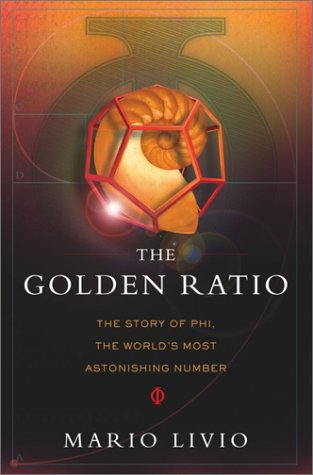The file `golden.m` inside the `assign1_exercises` folder contains two assignment statements that create two vectors that each store 10 measurements obtained from the arms of 10 very accommodating friends. The variable `hands` stores measurements of their hand length (in inches), in increasing order. The variable `forearms` stores the corresponding forearm lengths. These statements have a semi-colon at the end, so the values are not printed in the MATLAB Command Window when the code is executed. In this exercise, you will expand the `golden.m` code file in multiple steps. After completing each step, save the modified file and execute the new code by entering the command `golden` in the Command Window. To begin, open the `golden.m` file in the editor, and add comments to the top of the file with the names of you and your partner.

#### (a) Plot the data

Add a call to the `plot` function to display a graph of forearm length vs. hand length (i.e., plot hand length on the x axis and forearm length on the y axis). If the ratio of these two lengths is roughly the Golden Number Phi, how should your graph appear?

(Optional) If you add the following command after your call to the `plot` function, the values on the x and y axes will be plotted on the same scale, which means that the physical distance on the screen that is spanned by one unit on the x axis is the same physical distance spanned by one unit on the y axis:

`axis equal`

#### (b) Create a vector of forearm:hand ratios

Add a single assignment statement that creates a new vector of 10 ratios, obtained by dividing each forearm length stored in `forearms` by its corresponding hand length stored in `hands`.

#### (c) Plot the ratios

Use the `plot` function to display a graph of the ratios generated in Part (b). The ratios can be plotted on the y axis. Draw this graph in a new figure window by placing the `figure` command before the second call to the `plot` function. When you now execute your code, two figure windows will be drawn on top of one another and can be dragged apart with the mouse.

Important note: After executing your new code and viewing the results, close the figure windows before executing your code again! Having an excessive number of open windows can sometimes cause code to execute improperly. You can close each window by clicking on the close box in the upper right corner of the window. You can close all of the figure windows at once by executing the following command in the Command Window:

`>> close all`

#### (d) Calculate the mean ratio

Calculate the mean, or average value of the ratios computed in Part (b) and assign a variable to this mean value. Do not use the built-in `mean` function (although it's OK to use `mean` to check your answer). What are we hoping this mean value to be?

#### (e) Add the mean ratio to the graph

Using `plot` again, add a horizontal line to the graph that you created in Part (c), whose height is the mean ratio. Set the color of this line to be different from the default blue color of the graph of ratios. To add a second plot to an existing graph, place the following command before the next call to `plot`:

`hold on`

To create the coordinates of points to draw the horizontal line, keep in mind that the `plot` function only needs two points to draw a line! Also note that the names of variables can be placed inside an assignment statement that creates a vector of values, as illustrated in the following code:

```  num = 3   moreNums = [num 2*num 3*num]```

With a ruler, measure the length of your own forearm and hand (to see how these distances are determined, see the picture on The Human Hand & Foot page). Add two assignment statements to your code that each use the `input` function to ask the user to enter their forearm length and hand length, and assign each of the user's inputs to variables. Compute the ratio of the user's forearm to hand length, and assign this ratio to a new variable. Finally, draw a horizontal line on the graph with a new color, whose height is the ratio computed for the user's (your) arm.

After adding this final line, add the following statement to your code so that future graphs are not drawn on the same figure:

`hold off`

Use the functions `xlabel, ylabel` and `title` to add labels to the two graphs that you created.

Use the MATLAB function `text` to add a text label (e.g. 'My ratio') to the line that shows your ratio. `text` works like this:

`text(xpos, ypos,'your text here');`

You'll notice that there are some comments at the beginning of the code file that provide the file name, initial creator and date of creation, and a brief description of what the program does. Add a comment near the beginning that includes your name and the last date on which you modified the code. If you worked with a partner, include the name of your partner as well. Then add a few additional brief comments throughout the code that summarize groups of statements that implement each major task. For example, you might add a comment like "compute and display the mean ratio" prior to the sequence of statements that you added for Parts (d) and (e). Comments like this are helpful unto other people who read your code and also help you to remember what you did if you need to modify the code sometime in the (distant) future. Comments that appear at the beginning of a script file are printed by the `help` function:

`help golden`

## Exercise 2: Practice with conditional expressions

Create a new file in MATLAB called `conds.m` (that you will turn in). Make sure your Current Directory is set to the `assign1_exercises` folder when you save this code file.

In `conds.m`, first use MATLAB's `input` function to prompt the user for three pieces of information: 1) a numerical `month` (between 1 and 12), 2) a `day` (between 1 and 31) and 3) the user's name. Store each of these values in a variable (e.g. `month` is 7 (for July), `day` is 28 and `name` is 'Rosa'). To prompt the user for a string, provide a second input `'s'` when calling the `input` function:

``` name = input('Enter your name: ', 's');   % name is a string ```

Then write MATLAB expressions that correspond to the following:

1. Create a variable `valentine` that is true on February 14 and false otherwise.
2. Create a variable `cs112midterm` that is true on March 2 and April 23, and false otherwise.
3. Create a variable `springBreak` that is true between March 19 and March 29 (inclusive).
4. If `month` and `day` are your birthday, then print out a personalized birthday greeting such as "Happy Birthday to Stella!", otherwise print "Not Stella's birthday". The `disp` function can be used to print text that combines literal strings with variables whose value is a string:
```>> place = 'SCI 257';
>> disp(['class will be held in ' place ' today']);
class will be held in SCI 257 today```
5. If `month` is December, January or February, then print the lyrics of the first stanza of the song, "Frosty the Snowman" (on four separate lines), otherwise print a message of your choosing (this can be a single line).Frosty the Snowman was a jolly happy soul,    With a corncob pipe and a button nose and two eyes made out of coal.    Frosty the Snowman is a fairy tale they say,    He was made of snow but the children know how he came to life one day.
You can test your variables by inputting different values for `month ` and `day` and then seeing if your variables contain the correct values. Add comments containing the names of you and your partner, and the date, to your `conds.m` file and upload it with your other MATLAB files in your `assign1_exercises` folder, when turning in Assignment 1.

## Problem 1: The 2014 Winter Olympics are Finally Here!

 The 2014 Winter Olympic Games in Sochi are now behind us and the 2018 2018 PyeongChang Games may seem far away, but we can still try to get into the spirit by creating our own olympic symbol! Using the `plot` function and a little creativity, construct a variation on the olympic symbol similar to the figure shown below.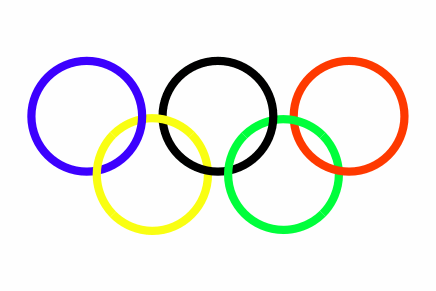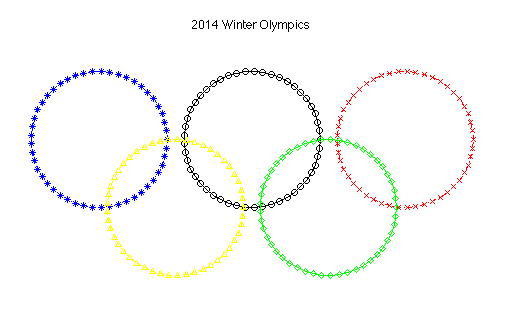The code for making this picture should be placed in a file named `olympics.m` that you create from scratch and store in a folder named `assign1_problems` that you also create. The easiest way to start a new code file is to type the `edit` command in the Command Window, followed by the first name of the file that you want to create:

`>> edit olympics`

A pop-up box will appear that tells you that the file `olympics.m` does not exist and asks if you want to create it. Click on the `Yes` button and an empty window for entering your code will appear in the Editor. Type a couple comments at the beginning of the file with the name of the file, your name, and the name of your partner if you are working in a pair, and you'll be ready to start working on creating your picture. Similar to what you did to complete the Golden Ratio exercise 1, it will help to proceed in stages.

### (1) Draw a single circle

To draw a circle, you can use the `plot` function to draw a curve that connects a set of equally spaced points around the circumference of the circle. The `x` and `y` coordinates of each point can be defined in polar coordinates as illustrated in the figure below. `r` is the radius of the circle and `a` is the angle between the x axis and the line connecting the origin to a point on the circle (shown in green). The coordinates of the point on the circle are given by the following expressions:

```x = r * cos(a)       y = r * sin(a)```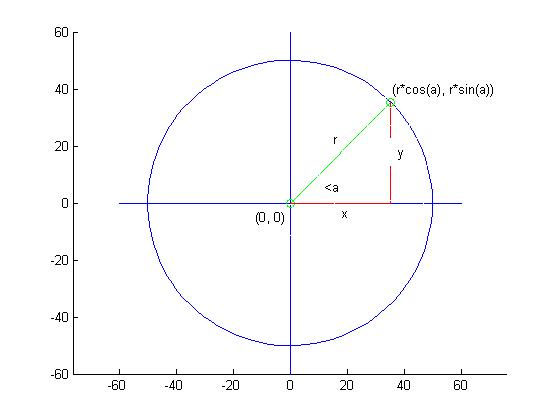The `sin` and `cos` functions require the input angle to be given in radians. Click here for a quick review of trigonometry and generating points around a circle. There is a special constant `pi` whose value is π (3.14159...) that is helpful for generating angles in radians. The input to the `sin` and `cos` functions can either be a single angle or a vector of angles. When a vector of angles is given, these functions generate a vector of sine and cosine values for all of the angles in the input vector.

With this background in mind, generate the x and y coordinates for points on the circle by first creating a vector of equally spaced angles from 0 to 2π, and then using the expressions above to compute the x and y coordinates from the vector of angles. The olympic symbol shown above was created using 50 equally spaced angles around the circle, but you can vary this number. You also need to specify a value for the radius of the circle. Draw the circle with the `plot` function and observe your results - it may look more like an oval than a circle! To make the curve appear as a true circle, add the `axis equal` command.

### (2) Draw four more circles

The circle shown above is centered at the origin, (0,0). To draw the remaining circles, shift the coordinates to a new center location when plotting. Be sure to add the `hold on` command before plotting the second circle, and the `hold off` command at the end of your code. Add color, linestyles and markers to each call to the `plot` function so that each circle is displayed with a different color, linestyle and marker. Set the range of values that are displayed on the axes so that there is white space all around the picture. This can be done by calling the `axis` function with an input that is a vector of four values corresponding to the minimum and maximum x values to plot, and the minimum and maximum y values:

`axis([xmin xmax ymin ymax])`

You can also turn off the axes around the picture with the following command:

`axis off`

When the axis is turned off, the background color reverts to the default gray color. You can set the background color to white with the following command:

`set(gcf, 'Color', 'w')`

`gcf` refers to the current figure window.

### (3) The finishing touches

Use the `title` command to add a title to the figure, and add some comments to the code that include the name of the file, the names of you and your partner, and the date at the beginning of the file, and describing what is done by the major parts of the code.

Note: For your final submission, you do not need to hand in a hardcopy of your picture, only a copy of your `olympics.m` code file.

## Problem 2: Star Light, Star Bright

 Ever wonder why some stars appear brighter than others? Astronomists have observed that the brightness of a star depends on both its size and temperature. This dependence is captured in a Hertzsprung-Russell (H-R) diagram such as the one shown below. The ratio between the luminosity of a star and the luminosity of the sun is plotted on the vertical axis and the temperature of the star in degrees Kelvin is plotted on the horizontal axis, with temperature decreasing from left to right. Each dot in the diagram represents a single star. In general, hotter stars appear brighter and lie along the main sequence. The cooler giants and supergiants appear very bright due to their large size, while the hot white dwarfs appear faint because of their tiny size.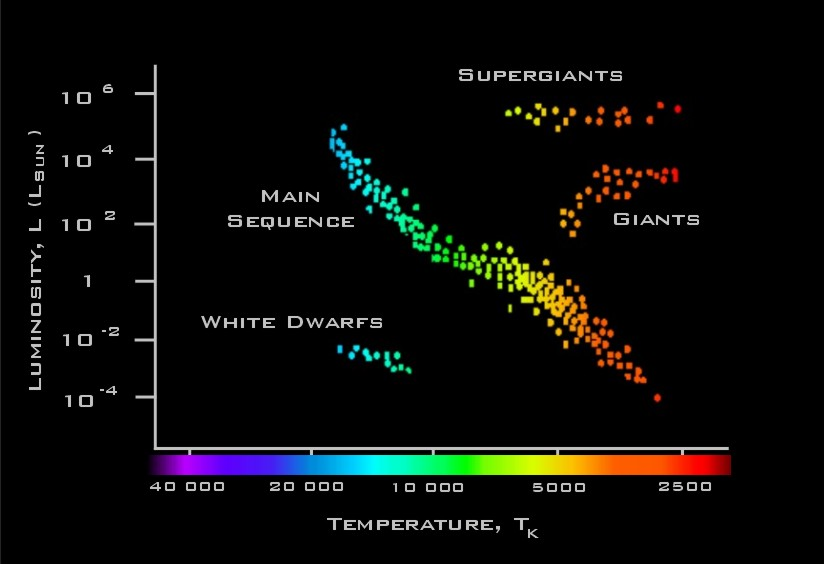Astronomists have modeled the relationship between luminosity, size and temperature using the following equation:

Lstar/Lsun = (Rstar/Rsun)2 (Tstar/Tsun)4

where Lstar/Lsun is the ratio between the luminosity of a particular star and the luminosity of the sun, and Rstar/Rsun and Tstar/Tsun are similar ratios for the size and temperature of the star relative to the sun. The following table provides data on the ratios Lstar/Lsun and Rstar/Rsun, and the temperature values for a set of stars. The aim of this problem is to determine how well the above model fits the observed data1.

 Sun Barnard'sStar EpsilonIndi Alioth Regulus Spica BetaCrucis Lstar/Lsun 1 0.0004 0.15 108 150 13400 34000 Rstar/Rsun 1 0.18 0.76 3.7 3.5 7.8 8 Temp (K) 5840 3130 4280 9400 13260 22400 28200

Write a MATLAB script named `stars.m` that creates an H-R diagram from the above data and model, and quantifies the deviation between the two. Your `stars.m` code file should be placed inside your `assign1_problems` folder. If your are working with a partner, add comments to the top of the code file with the names of both partners.

In your script, create a single figure that contains two line plots corresponding to the data and model predictions. Display the two plots with a different color and marker. Use the same temperature values for the two plots. In the case of the luminosity ratio, use values from the above table for one plot, and values computed by the above model for the second plot. Your figure should resemble the plots of the model and data for a cooling cup of coffee shown in the last slide from lecture #3. Create vectors to store the data from the table, the temperature ratios Tstar/Tsun and the predicted values of Lstar/Lsun. Add axis labels, a title and legend to your figure, and use the `axis` command to set the range of values on the axes so that there is some space between the axes and plots. Remember that in an H-R plot, the temperature values are displayed in decreasing order on the horizontal axis. This can be accomplished in MATLAB by added the following statement:

`set(gca, 'XDir', 'reverse')`

The data and model predictions will appear to be very close, but let's quantify the fit. A common measure of deviation between a set of data values and the predictions of a model is the root mean square error (rms error) defined by the following expression: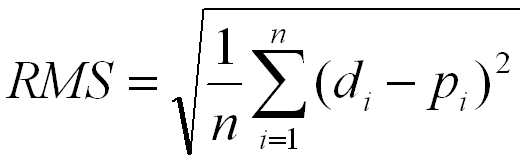where di is the ith data value (e.g. the luminosity ratio measured for the ith star), pi is the predicted ith value (e.g. the luminosity ratio predicted from the model, for the ith star), and n is the total number of data samples. The rms error captures the average difference between the measured and predicted values. Add code to your `stars.m` script to calculate the rms error and print its value. Note that all of the arithmetic operations needed to compute the rms error can be combined into one MATLAB statement!

Note: Again, for your final submission, you do not need to hand in a hardcopy of your picture, only a copy of your `stars.m` code file.